﻿

### The annihilator ideal graph of a commutative ring

#### Abstract

Let$R$ be a commutative ring with nonzero identity and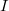$I$ be a proper ideal of$R$. The annihilator graph of$R$ with respect to$I$, which is denoted by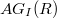$AG_{I}(R)$, is the undirected graph with vertex-set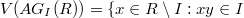$V(AG_{I}(R)) = \lbrace x\in R \setminus I : xy \in I\$ for some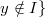$\ y \notin I \rbrace$ and two distinct vertices$x$ and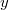$y$ are adjacent if and only if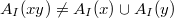$A_{I}(xy)\neq A_{I}(x) \cup A_{I}(y)$, where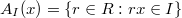$A_{I}(x) = \lbrace r\in R : rx\in I\rbrace$. In this paper, we study some basic properties of$AG_I(R)$, and we characterise when$AG_{I}(R)$ is planar, outerplanar or a ring graph. Also, we study the graph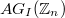$AG_{I}(\mathbb{Z}_{n})$, where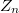$Z_n$ is the ring of integers modulo$n$.

DOI Code: 10.1285/i15900932v36n1p1

Keywords: Zero-divisor graph; Annihilator graph; Girth; Planar graph; Outerplanar; Ring graph

Full Text: PDF